# Drug interaction modeling based on Loewe additivity following Harbron’s approach

#### November 9, 2018

This vignette provides an overview of the drugCombo R package functionality and capabilities.

# Introduction and Methods

Combinations of different biological active agents are of interest in several fields and often provide therapeutic advantages over single agents. Drug combinations are generally described as being synergistic or antagonistic. The Loewe additivity model (Loewe 1953) is one of the most commonly used models to quantify a zero-interactive state for the combination of two drugs: $\frac{d_1}{D_{1}} + \frac{d_2}{D_{2}} \begin{cases} = 1, & \text{additivity} \\ <1, & \text{synergy} \\ >1, & \text{antagonism} \end{cases}$ where $$d_1$$ and $$d_2$$ represent the doses of the two compounds that in combination produce an effect $$y$$, and $$D_1$$ and $$D_2$$ represent the doses of the two compounds that produce the same effect $$y$$ when given as a monotherapy.

Harbron (2010) introduced a flexible framework to assess in vitro synergy by fitting a hierarchy of interaction index models to drug combination data using the Loewe additivity model. The interaction index $$\tau$$ is defined by the sum of the two dose fractions in the Loewe additivity model. Harbron (2010)’s method roughly consists of the following three steps:

• Fitting a dose-response model to the monotherapy data.
• Estimating the interaction index $$\tau$$ based on Loewe model.
• Comparing several parametric models for the interaction index $$\tau$$.

The R package drugCombo is building upon and extending the methods described in Harbron (2010). The main extensions are:

• Support for flexible (formula-like) specification for the interaction index ($$\tau$$).
• Support for “2-stage” estimation in addition to “1-stage” estimation, including a bootstrap procedure to compute confidence intervals.

Under the Loewe model, when one of the two drugs exhibits a partial monotherapy response, this drug is assumed not to contribute to combination effects greater than this maximum response. When both drugs have a partial monotherapy response and the observed combination effect is greater than the upper asymptote of both of the monotherapy curves, the interaction index $$\tau=0$$ (Harbron 2010). If the second compound is inactive as a monotherapy, the Loewe model reduces to the highest single agent model so that under additivity the response of the combination is completely determined by the dose of the first compound.

## 1-stage versus 2-stage estimation

Harbron (2010) used a 1-stage estimation approach, where the interaction index $$\tau$$ and monotherapy dose-response parameters are estimated simultaneously using the pooled monotherapy and combination data. This may be counterintuitive as monotherapy parameter estimates could change each time a different model for the interaction index is considered. Alternatively in a 2-stage estimation approach, as proposed by Zhao et al. (2012), monotherapy parameters are estimated first, and secondly the interaction index is estimated while keeping the monotherapy parameter estimates fixed. We propose a non-parametric bootstrap procedure to account for the uncertainty of the monotherapy estimation in the first stage.

Simulations indicated that there is little difference between 1-stage and 2-stage estimation results when the interaction index model is correctly specified. However, if the model for $$\tau$$ is not correctly specified, both approaches may produce biased interaction estimates (similar RMSE) while monotherapy parameters are only biased in the one-stage approach (Nazarov and Goeyvaerts, “One and two-stage modelling approaches for assessing synergy in Harbron’s framework”, Non-Clinical Statistics Conference 2016, Cambridge, UK).

## Study design and data format

The package works with data in a “long” format, consisting of measurements of the effect at different dose combinations of the two drugs. Required column names are d1, d2 for the doses and effect for the effect. Additional variables may be present in the data. Two example datasets are included in the package, and can be accessed with:

data("checkerboardData", package = "drugCombo")
data("rayData", package = "drugCombo")

These datasets represent two common choices of experimental design:

• In a “checkerboard” (or grid) design, a certain set of dose levels is chosen for each compound, and then all possible pairwise dose combinations are tested.
• In a “ray” (or fixed-ratio) design, dose combinations are chosen in such a way that dose ratios are constant along the “rays”.

# Monotherapy Fitting

Monotherapy dose-response curves for each of the two compounds are assumed to follow the Hill equation with common baseline value (at dose 0):

$y\left(d\right) = b + \dfrac{m - b}{1 + \left(\frac{e}{d}\right)^{|h|}}$

where $$y$$ is the response (or effect), $$d$$ is the dose (or concentration) of the compound, $$h$$ is the Hill’s coefficient and $$b$$ and $$m$$ are respectively baseline and maximum response for that compound. Lastly, $$e$$ stands for EC50, the dose level of the compound needed to attain the midpoint effect, i.e. $y\left(e\right) = b + \frac{m - b}{2}$

Note that $$m > b$$ if and only if the response is increasing with the dose of the compound. If the response is decreasing, then $$m < b$$.

This monotherapy equation is estimated for both compounds with the constraint that $$b$$, the baseline level, is shared across compounds. This baseline level is denoted by b in the parameter vector. Additionally, m1 and m2 in the parameter vector stand for estimates of maximal responses $$m_{1}$$ and $$m_{2}$$, respectively, whereas h1 and h2 are Hill’s coefficients (slope) of the monotherapy curve for each compound. Lastly, e1 and e2 are log-transformed inflection points, i.e. e1 $$= \log\left(e_{1}\right)$$ and e2 $$= \log\left(e_{2}\right)$$.

The estimations is performed with the fitMarginals function (imported from the BIGL package) as follows:

gridData <- checkerboardData[checkerboardData\$exp == 1, ]  # subset the data

monoGrid <- fitMarginals(gridData, fixed = c(b = 1))
monoRay <- fitMarginals(rayData)

The fixed argument allows to fix specific monotherapy dose-response parameters.

A MarginalFit object is returned, which has summary and plot methods available:

summary(monoGrid)
## Formula: effect ~ fn(h1, h2, b = 1, m1, m2, e1, e2, d1, d2)
## Transformations: No
##
##                  Compound 1 Compound 2
## Slope                 0.656      3.494
## Maximal response      0.043      0.014
## log10(EC50)           0.991      0.491
##
## Common baseline at: 1
plot(monoGrid)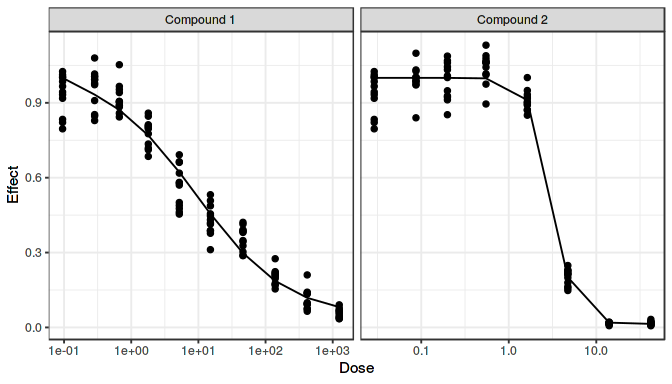summary(monoRay)
## Formula: effect ~ fn(h1, h2, b, m1, m2, e1, e2, d1, d2)
## Transformations: No
##
##                  Compound 1 Compound 2
## Slope                 0.801      0.806
## Maximal response    -76.338   -198.053
## log10(EC50)           0.519     -0.380
##
## Common baseline at: 3975.739
plot(monoRay)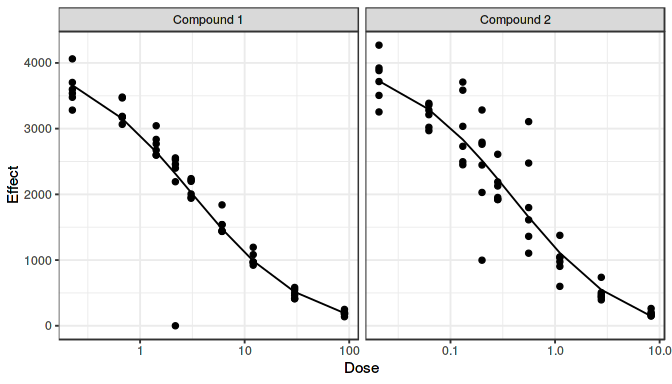The fit of the monotherapy model i.e. the fitMarginals object, is used further as starting values in a 1-stage estimation approach or provides the actual values for the monotherapy parameters in case of a 2-stage estimation approach.

# Tau Estimation

In Harbron (2010)’s framework, the model that is fitted to the data can be written as:

$\frac{d_1}{D_1}+\frac{d_2}{D_2} = \begin{cases} 1, & d_1 = 0~\text{or}~d_2 = 0 \\ \tau_{(i)}, & d_1 > 0~\text{and}~d_2 > 0 \end{cases},$ where $$d_1$$ and $$d_2$$ represent the doses of the two compounds that in combination produce an effect $$y$$, $$D_1$$ and $$D_2$$ represent the doses of the two compounds that produce the same effect, $$y$$, and $$\tau_{(i)}$$ provides a mechanism for allowing the degree of synergy to vary across the experimental space.

Thus the response $$y$$ is expressed as an implicit function of the doses $$d_1, d_2$$, monotherapy parameters $$\phi$$ and interaction indices $$\tau_{(i)}$$, $$y = f(d_1, d_2, \phi, \tau_{(i)})$$.

Notation-wise, we routinely call interaction indices as “tau” in the text.

## Tau models

Different parametric models are supported for the interaction index $$\tau$$. The package contains a set of pre-defined models that were proposed by Harbron (2010) and Zhao et al. (2012). Further, the user can use a formula object to specify any parametric model suitable for the data at hand. The package thus allows full flexibility when modelling the interaction between compounds.

### Pre-defined models

The pre-defined models are defined below: "additive", "uniform", "linear1", "linear2", "separate1", "separate2", "separate12", "zhao". All models except for "zhao" follow the Harbron (2010) approach, and are appropriate for checkerboard designs.

Model Formula Description Number of parameters
additive $$\tau_{(i)} = 1$$ additivity 0
uniform $$\tau_{(i)} = \tau$$ one overall value for tau 1
linear1 $$\tau_{(i)} = \tau_1+\tau_2\log_{10}(d_1)$$ linear dependency on log10 dose of the first compound 2
linear2 $$\tau_{(i)} = \tau_1+\tau_2\log_{10}(d_2)$$ linear dependency on log10 dose of the second compound 2
separate1 $$\tau_{(i)} = \sum_{\{d_1\}}\tau_{d_1}I_{(d_1)}$$ different tau for each dose of the first compound number of doses $$d_1$$
separate2 $$\tau_{(i)} = \sum_{\{d_2\}}\tau_{d_2}I_{(d_2)}$$ different tau for each dose of the second compound number of doses $$d_2$$
separate12 $$\tau_{(i)} = \sum_{\{d_1,d_2\}}\tau_{(d_1,d_2)}I_{(d_1, d_2)}$$ different tau for each
combination of doses of the two compounds
number of combinations of doses
$$d_1$$ and $$d_2$$
zhao $$\tau_{(i)}=\exp(\tau_1+\tau_2\log(d_1)+\\ \ +\tau_3\log(d_2)+\tau_4\log(d_1)\log(d_2)+\\ \ +\tau_5(\log(d_1))^2 + \tau_6(\log(d_2))^2)$$ quadratic response surface model following Zhao et al. (2012) 6

### Model formula

The interaction index can also be modeled by means of a user-specified function of doses and/or other variables available in the data: $$\tau_{(i)} = f(d_1, d_2, ...)$$. The package supports two types of formulas:

• “symbolic”, following R model formula specification rules, for example, ~ log10(d1)
• “literal”, for example, ~ tau1 + tau2*log10(d1) for an equivalent to the above symbolic formula. In this case, the interaction indices to be estimated should be named tau1, tau2, etc.

Some tau model functions can be defined by either of the two ways (and this would lead to identical results), while for some other functions one or another way may be more convenient.

Apart from the doses, other variables present in the data can be also used in the formulas. For example, in a ray design experiment, a formula could be ~ 0 + ray to allow interaction indices to vary across rays (here ray is a character or a factor variable in the data).

Note that the formulas above are used only for the interaction indices for combinations of doses, while for the monotherapies, tau is assumed to be equal to 1. Therefore, continuous models may entail discontinuities in the interaction index when $$d_1$$ and $$d_2$$ approach 0.

## fitModel function

The function fitModel allows to fit the chosen interaction index model to the data. The estimated parameters are the interaction indices tau1, tau2, etc., and in the 1-stage estimation approach also the monotherapy dose-response parameters.

The required arguments are data and either a model or tauFormula for a pre-defined or user-specified model for the interaction index, respectively. The mono argument requires a fitMarginals object and determines the starting values in a 1-stage estimation approach or provides the actual values for the monotherapy parameters in case of a 2-stage estimation approach. If the mono argument is not provided, default monotherapy fitting (without constraints) is performed. Constraints on all parameters (monotherapy and tau) can be defined with the fixed argument that works in the same way as for the fitMarginals function. Further arguments for the interaction indices allow estimation on the log-scale (tauLog, default is FALSE) and specifying starting values (tauStart). Choice of 1-stage or 2-stage estimation is governed by the stage argument (default is 1-stage). A situation when one compound is inactive as monotherapy is supported via inactiveIn argument. It can take values 1, 2 or 0 (default, when both compounds are active).

Some examples are shown below to illustrate the use of the fitModel function.

For a checkerboard design, illustrating the pre-defined model, symbolic and literal formula for the same model with a linear dependency on log10 dose of the first compound:

fitLin1 <- fitModel(data = gridData, mono = monoGrid, model = "linear1")
## fitLin1b <- fitModel(gridData, monoGrid, tauFormula = ~ log10(d1))
## fitLin1c <- fitModel(gridData, monoGrid, tauFormula = ~ tau1+tau2*log10(d1))

For a checkerboard design, illustrating 2-stage estimation:

fitLin1St2 <- fitModel(gridData, monoGrid, model = "linear1", stage = 2)

Apart from continuous models, such as the linear model, also discrete models can be fitted such as:

fitSep1 <- fitModel(gridData, monoGrid, model = "separate1", tauLog = TRUE)
## fitSep2 <- fitModel(gridData, monoGrid, model = "separate2", tauLog = TRUE, fixed = c(b = 1, h2 = 3.494))

where a different tau is estimated for each dose of the first and second compound, respectively. Note that in the second model, the monotherapy Hill slope for drug 2 was fixed.

A situation with one compound being inactive can be modelled with:

fitInactive1 <- fitModel(gridData, model = "uniform", inactiveIn = 1)
summary(fitInactive1)
##
## Formula: effect ~ tauModelFormula(d1 = d1, d2 = d2, h2 = h2, e2 = e2,
##     b = b, m2 = m2, tau1 = tau1, formula = ~1, tauSpec = "symbolic",
##     tauLog = FALSE, inactiveIn = 1)
##
## Parameters:
##      Estimate Std. Error t value Pr(>|t|)
## h2    1.58457    0.28143   5.630 2.36e-08 ***
## b     0.54536    0.01605  33.982  < 2e-16 ***
## m2   -0.02117    0.02251  -0.940    0.347
## e2    1.87772    0.29886   6.283 5.04e-10 ***
## tau1  0.24414    0.07397   3.300    0.001 **
## ---
## Signif. codes:  0 '***' 0.001 '**' 0.01 '*' 0.05 '.' 0.1 ' ' 1
##
## Residual standard error: 0.2773 on 955 degrees of freedom
##
## Number of iterations to convergence: 21
## Achieved convergence tolerance: 1.49e-08

The monotherapy parameters of the inactive compound are not estimated in that case.

For a ray design, illustrating the model with a different interaction index per ray:

fitRay <- fitModel(rayData, monoRay, tauFormula = ~ 0+ray)

More complex models can be fitted as well:

fitExp1 <- fitModel(gridData, monoGrid, tauFormula = ~ exp(tau1+tau2*log(d1)), stage = 1)
fitExp1St2 <- fitModel(gridData, monoGrid, tauFormula = ~ exp(tau1+tau2*log(d1)), stage = 2)
fitZhao <- fitModel(gridData, monoGrid, model = "zhao", tauStart = 0)

### Convergence issues

Note that for complex models with many parameters, the fitModel function might not lead to convergence. This means that the model is ill-defined or not estimable from the data at hand. This can also happen when the monotherapy data does not follow the assumed log-logistic shape. Sometimes fitting tau on the log scale (with tauLog = TRUE) may help to achieve better convergence. In case of problems with convergence, using nls’s argument trace = TRUE can be useful to detect which parameters cause these problems. In case of identifiability issues, one might consider fixing certain model parameters with due caution.

## Diagnostic plots

The fitModel function returns a HarbronFit object, which is an nls object with extra elements. The summary method from nls can be used to have an overview of the model fit:

summary(fitExp1)
##
## Formula: effect ~ tauModelFormula(d1 = d1, d2 = d2, h1 = h1, h2 = h2,
##     e1 = e1, e2 = e2, b = 1, m1 = m1, m2 = m2, tau1 = tau1, tau2 = tau2,
##     formula = ~exp(tau1 + tau2 * log(d1)), tauSpec = "literal",
##     tauLog = FALSE, inactiveIn = 0)
##
## Parameters:
##        Estimate Std. Error t value Pr(>|t|)
## h1   -0.7036859  0.0146186 -48.136   <2e-16 ***
## h2    3.6457807  0.1208226  30.175   <2e-16 ***
## m1    0.0654409  0.0053805  12.163   <2e-16 ***
## m2   -0.0004407  0.0027345  -0.161    0.872
## e1    2.4154631  0.0406364  59.441   <2e-16 ***
## e2    1.0929747  0.0232194  47.072   <2e-16 ***
## tau1 -0.2881457  0.0240087 -12.002   <2e-16 ***
## tau2 -0.1924050  0.0076270 -25.227   <2e-16 ***
## ---
## Signif. codes:  0 '***' 0.001 '**' 0.01 '*' 0.05 '.' 0.1 ' ' 1
##
## Residual standard error: 0.04751 on 952 degrees of freedom
##
## Number of iterations to convergence: 10
## Achieved convergence tolerance: 1.49e-08

The plot method is defined and allows to display different types of diagnostic plots depending on the which argument:

• nls, default nlme::plot.nls plots.
plot(fitExp1)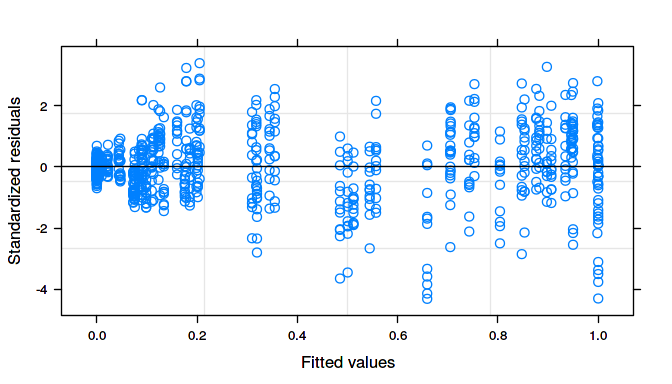• 2d, a ‘slice’ plot with fitted response overlaid on top of the observed data. This option allows to choose which ‘side’ to use for the x-axis: d1, d2, or total dose, the latter is useful for ray designs.
plot(fitExp1, which = "2d", side = "d1")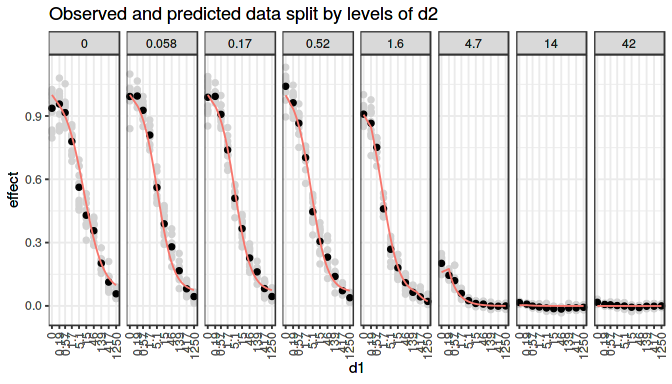plot(fitRay, which = "2d", side = "total")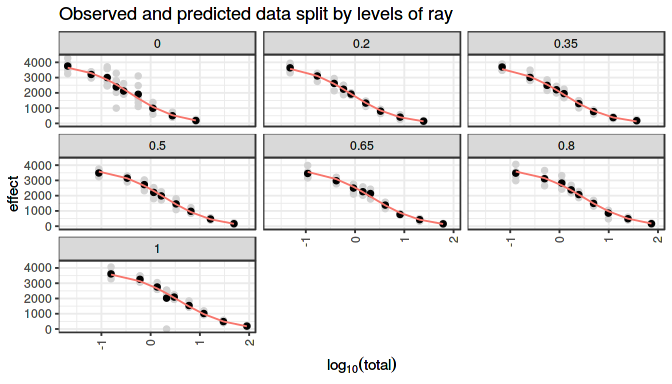• 3d, a 3d plot with fitted response surface overlaid on top of the observed data.
plot(fitExp1, which = "3d", widget = TRUE)

In addition, a graphical comparison can be shown when two models are fitted to the same data, e.g. comparing 1-stage and 2-stage estimation:

plot(fitExp1, fitExp1St2, modelNames = c("1-stage", "2-stage"))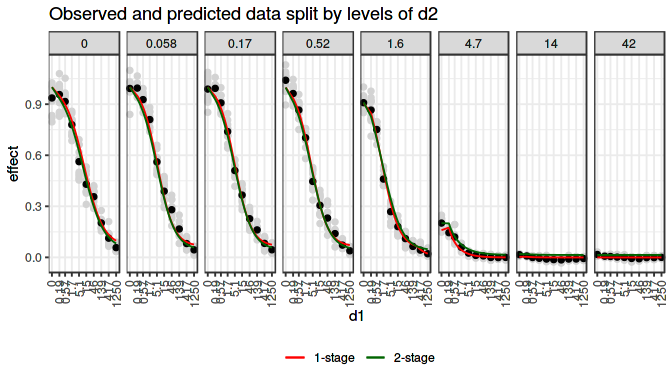## Tau graphical display

The getTauSurface function can be used to retrieve estimates of the interaction index at the tested dose combinations or for the whole experimental range (in case of a continuous tau model). The function provides point estimates for $$\tau$$ with corresponding standard errors and pointwise 95% confidence intervals. By default, asymptotic Wald-type confidence intervals are computed, but non-parametric bootstrap-based confidence intervals are available as well (with method = "boot"). Note that typically a large number of bootstrap replicates is needed to compute confidence intervals. In the examples below, only few iterations are used to avoid large computational time. Resampling type can be specified with resampling argument, which can take values “all” (default) for resampling from the whole data, “mono” for separate resampling of the monotherapy and combination data, and “stratified” for resampling by dose combinations. Note, that the latter option requires replicate measurements.

tauSurface1 <- getTauSurface(fitExp1, method = "default")
tauSurface1b <- getTauSurface(fitExp1, method = "boot", niter = 5)

tauSurface2 <- getTauSurface(fitExp1St2, method = "default") 
## Warning in getTauSurface(fitExp1St2, method = "default"): Confidence
## intervals computed don't take into account error propagation from the 1st
## stage (mono). Please use method = 'boot' for this.
tauZhao <- getTauSurface(fitZhao)

For the 2-stage approach, the Wald-type confidence intervals do not take into account the uncertainty of the monotherapy estimation in the first stage, and therefore it is recommended to use the bootstrap-based confidence intervals instead.

tauSurface2b <- getTauSurface(fitExp1St2, method = "boot", niter = 5)

The getTauSurface function returns a tauSurface object, which has plot, contour, print and unique methods defined. The plot method provides “2d” or “3d” plots of the estimated interaction indices.

plot(tauSurface1)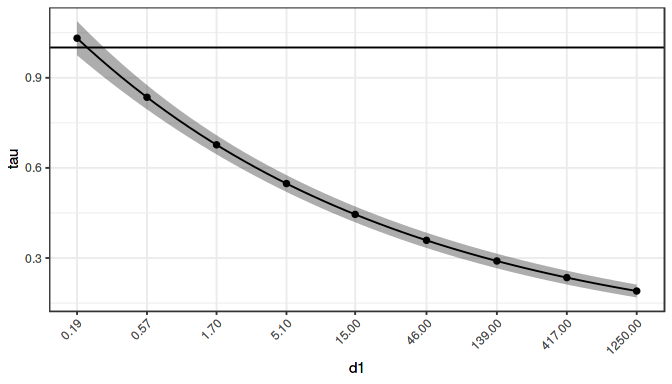plot(tauSurface1, which = "3d", widget = TRUE)